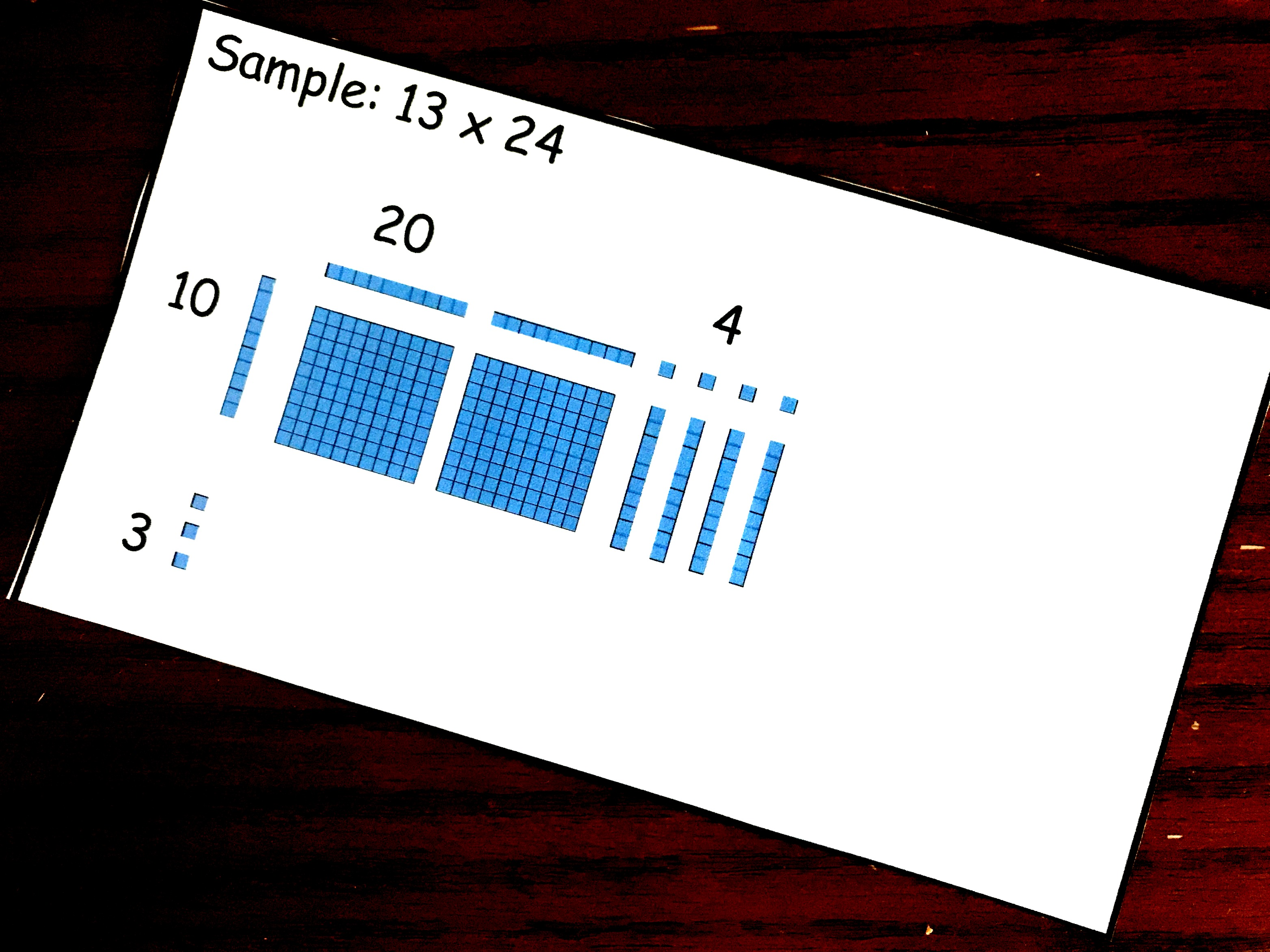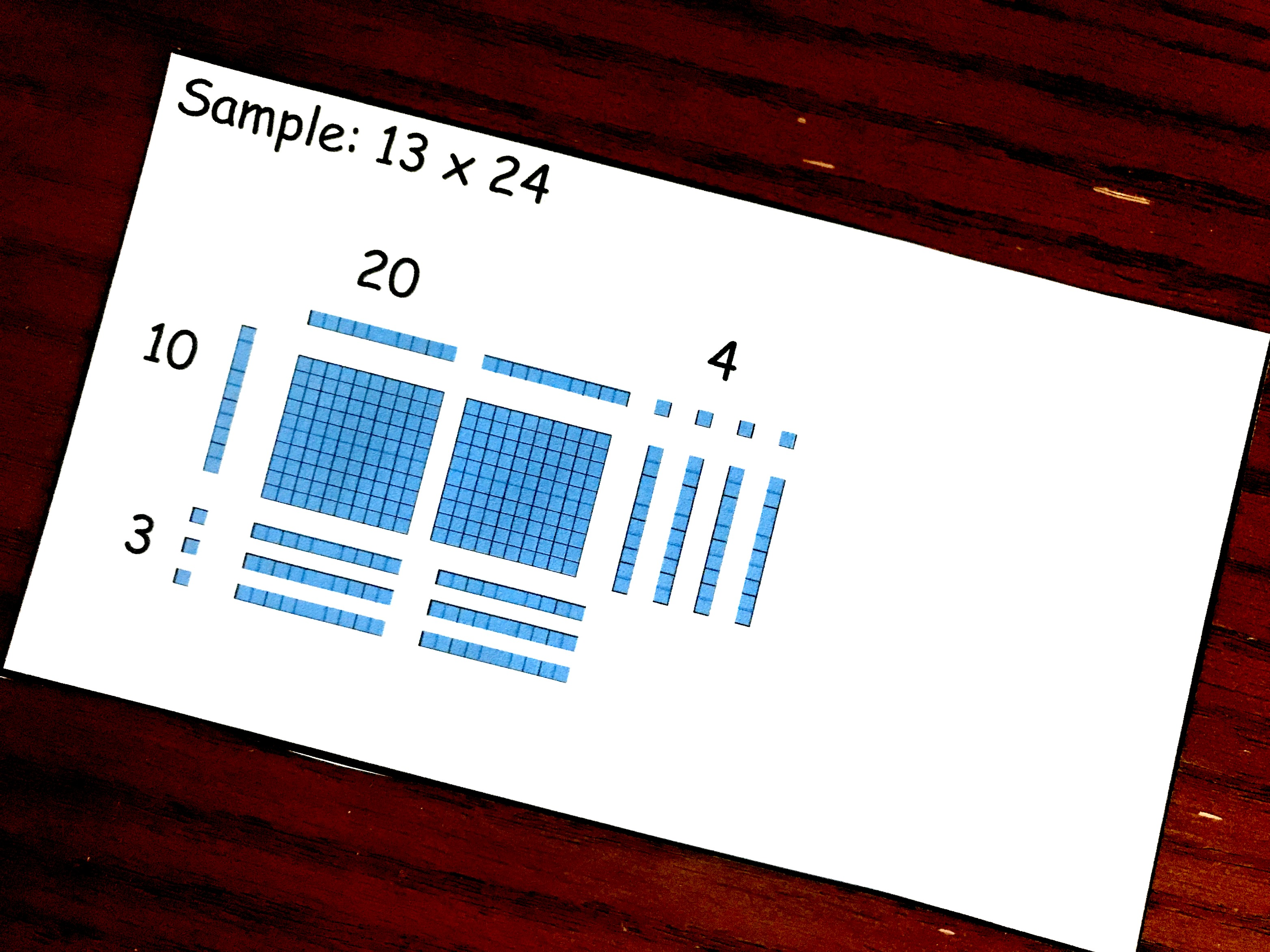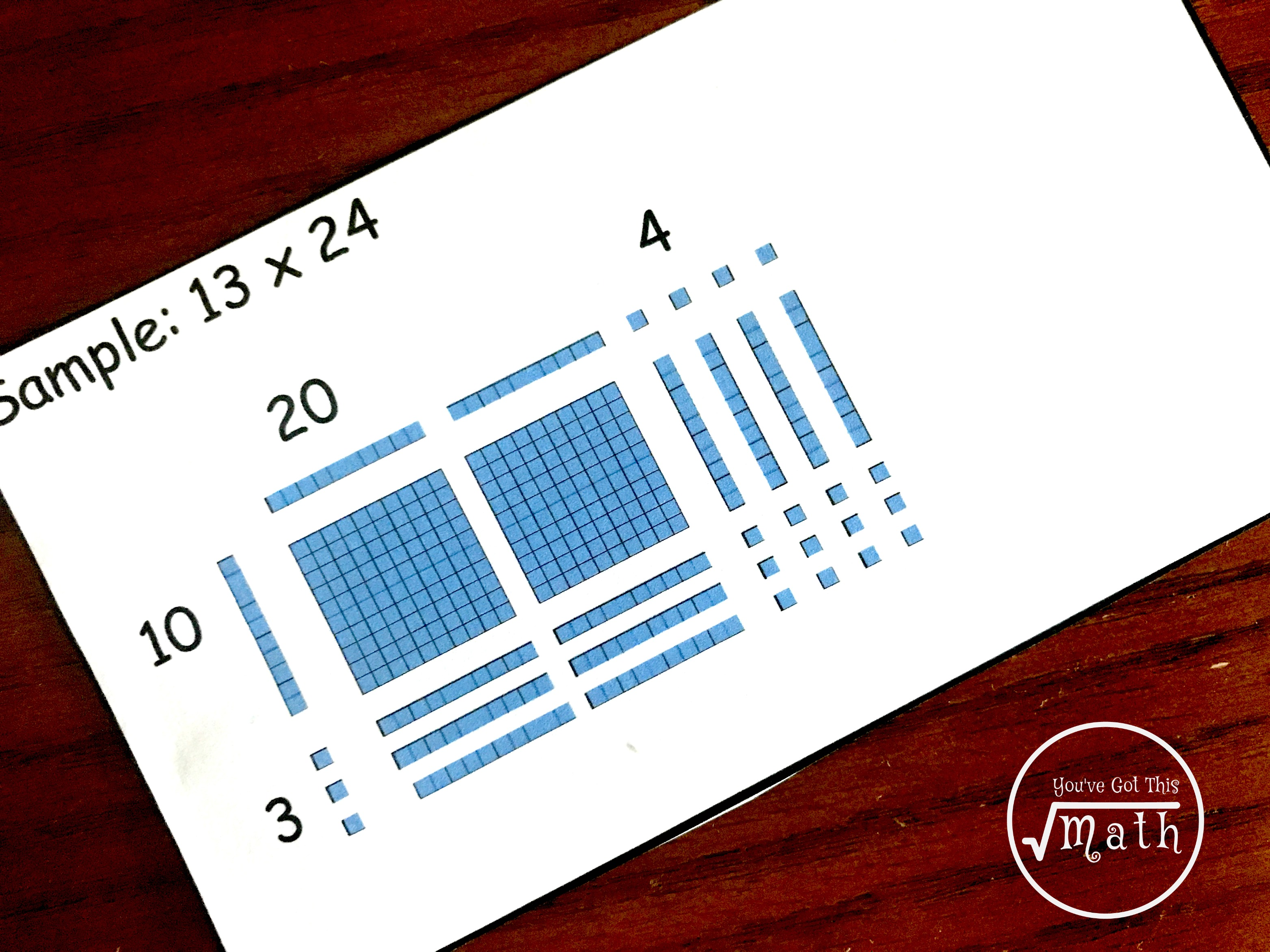Home » Math » How to Teach Large Digit Multiplication Using Arrays and Base Ten Blocks

How to Teach Large Digit Multiplication Using Arrays and Base Ten Blocks

Multiplication Using Arrays and Base Ten Blocks is a great way to demonstrate why methods such as partial products and area model work! And it is hands on.This post may contain Amazon links and links to other bloggers. There is no extra cost to you, but if you choose to purchase, I receive a small percentage. This goes to help with the upkeep of You’ve Got This and helps feed my family.

So today is part three of our study on different ways to teach multiplication. If you are wondering about why I like teaching different ways you can check out my last two posts about teaching partial products multiplication or the area model version.

Today we are going to pull out our base ten blocks and teach two digit multiplication by two digit multiplication using arrays. But it could easily be used on two digit by three digit or three digit by three digit multiplication. This “method” is very similar to area model. It would be easy to teach them together are around the same time.

Step One in Multiplication Using Arrays and Base Ten Blocks

Step one is to once again write out the factors in expanded form. One going across the page, and one going down the page.

Step Two: Create an Array with Base Ten Blocks

Moving on to step two, you get to create an outline of the array using base ten blocks that match the expressions you just created.

Here is an example of how to do this using the problem 24 x 38

1. The first number in the first expression is 20…so we place two longs going across the top of the page.

2. Since the second number is 4….so we place four cubes next to our two longs.

3. Now we move onto the first number in the second expression is 30….so we place three longs on the left going down.

4. Finally, we look at the second number which is 8….so we place eight ones next to our three longs.

STEP 3 – Make the Array

Next, you simply fill in the array using as few blocks as possible.

(If you have already taught multiplying using area model, this is a great place to make the connections. Have the children look back at the expanded form expressions. Have them multiply 30 x 20. When they see that this equals 600, they will know that they need to grab six flats.)

20 x 10  = 200 (place two flats down)10 x 4 = 40 (place 4 longs down)20 x 3 = 60 (place 6 longs in array)4 x 3 = 12 (place 12 cubes in array)Step 4 – Add up base ten blocks in Array

To finish up,  all you have to do is add up the base ten blocks that were used in the array.

200 +  40 +   60 +  12 = 312

Now I wouldn’t recommend this as a way to solve problems all the time….but it is a great place to let children explore with manipulatives and actually see why area model multiplication and even partial products multiplication work.

Enjoy!!!

Get Step by Step instructions on how to teach partial productsDownload your Step by Step Instructions on How to Teach Area ModelUsing LatticeTry teaching division using arrays.